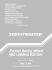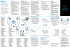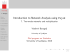# High-Performance Implementations on the Cell Broadband Engine

## Transcription

High-Performance Implementations on the Cell Broadband Engine
```High-Performance Implementations on the Cell
Joppe W. Bos
Laboratory for Cryptologic Algorithms, EPFL,
Lausanne, Switzerland
1 / 35
Outline
Project 1: 112-bit prime field ECDLP
Project 2: Fast arithmetic modulo a Mersenne number in ECM
2 / 35
3 / 35
Cell Availability
Speed
#SPEs
Memory
Price
Power
Compatibility
PS3
slim
3.2GHz
6
≈256MB
\$299.99
250W
PSOne
PS3
discontinued
3.2GHz
6
≈256MB
\$100 – \$300
280W
PSOne, Linux
PCIe
2.8GHz
8
4GB
≈ \$8k
210W
Linux
QS22?
3.2GHz
16
≤32GB
\$10k – \$14k
230W
Linux
? IBM PowerXCell 8i processor, offering five times the double precision
performance of the previous Cell/B.E. processor.
4 / 35
Cell architecture, the SPEs
The SPEs contain
a Synergistic Processing Unit (SPU)
SIMD operations
Dual pipeline (odd and even)
In-order processor
256 KB of fast local memory (Local Store)
Memory Flow Controller (MFC)
Direct Memory Access (DMA) controller
Handles synchronization operations to the other SPUs and the PPU
DMA transfers are independent of the SPU program execution
5 / 35
SPU registers
•
•
•
Byte:
Half-word:
Word:
16 × 8-bit SIMD
8 × 16-bit SIMD
4 × 32-bit SIMD
6 / 35
SPU registers
•
•
•
Byte:
Half-word:
Word:
16 × 8-bit SIMD
8 × 16-bit SIMD
4 × 32-bit SIMD
Theoretical performance of 16 × 3.2 · 109 = 51.2 billion 8-bit integer
operations per second.
6 / 35
Special SPU instructions
All distinct binary operations f : {0, 1}2 → {0, 1} are present.
Furthermore:
shuffle bytes
or across
average of two vectors
select bits
carry/borrow generate
only
but,
count ones in bytes
gather lsb
sum bytes
multiply and subtract
16 × 16 → 32-bit multiplication
16 × 16 + 32 → 32-bit multiply-and-add instruction
7 / 35
Special SPU instructions
All distinct binary operations f : {0, 1}2 → {0, 1} are present.
Furthermore:
shuffle bytes
or across
average of two vectors
select bits
carry/borrow generate
count ones in bytes
gather lsb
sum bytes
multiply and subtract
only 4-way SIMD 16 × 16 → 32-bit multiplication
but, 4-way SIMD 16 × 16 + 32 → 32-bit multiply-and-add instruction
7 / 35
SPU pipelines and latencies
Instruction class
Branch hints
Single-precision floating point
Double-precision floating point
Floating point integer
Shuffle
Simple fixed-point
Word rotate and shift
Latency
6
15
6
13∗
7
4
2
4
Pipeline
Odd
Odd
Even
Even
Even
Odd
Even
Even
One odd and one even instruction can be dispatched per clock cycle.
Challenge to the programmer (or compiler).
8 / 35
Considerations
Branching
No “smart” dynamic branch prediction
Instead “prepare-to-branch” instructions to redirect instruction prefetch
to branch targets
Memory
The executable and all data should fit in the LS
Or perform manual DMA requests to the main memory (max. 214 MB)
Instruction set limitations
16 × 16 → 32 bit multipliers (4-SIMD)
Challenge
One odd and one even instruction can be dispatched per clock cycle.
9 / 35
LACAL setup
Physically in the cluster room:
190 PS3s
6 × 4 PS3s in the PlayLaB
(attached to the cluster)
5 PS3 in our offices for
programming purposes
⇒ 219 PS3s in total.
10 / 35
11 / 35
Outline
Project 1: 112-bit prime field ECDLP
Joppe W. Bos, Thorsten Kleinjung, Arjen K. Lenstra, On the Use of the Negation Map in the Pollard Rho
Method, Algorithmic Number Theory (ANTS) 2010, volume 6197 of LNCS, pages 67–83, 2010
Joppe W. Bos, High-Performance Modular Multiplication on the Cell Processor, Arithmetic of Finite Fields
(WAIFI) 2010, volume 6087 of LNCS, pages 7-24, 2010
Joppe W. Bos, Marcelo E. Kaihara, Peter L. Montgomery, Pollard rho on the PlayStation 3, Handouts of
SHARCS 2009, pages 35-50
Project 2: Fast arithmetic modulo a Mersenne number in ECM
12 / 35
The ECDLP
The setting:
E is an elliptic curve over Fp with p prime.
P ∈ E (Fp ) a point of (prime) order n.
Q = k · P ∈ hPi.
Problem: Given E , p, n, P and Q what is k?
13 / 35
ECDLP Parameters
Certicom Challenge
Solve the ECDLP for EC over Fp (p odd prime) and F2m .
109-bit prime challenge solved in November 2002 by Chris Monico
Required time: 4000-5000 PCs working 24/7 for one year.
Next challenge is an EC over an 131-bit prime field
The 131-bit challenge requires 2000 times the effort of the 109-bit
14 / 35
ECDLP Parameters
ECC Standards
Standard for Efficient Cryptography (SEC),
SEC2: Recommended Elliptic Curve Domain Parameters
Prime fields bit length: { 112, 128, 160, 192, 224, 256, 384, 521 }
Wireless Transport Layer Security Specification
Prime fields bit length: { 112, 160, 224 }
Digital Signature Standard (FIPS PUB 186-3)
Prime fields bit length: { 192, 224, 256, 384, 521 }
15 / 35
ECDLP Parameters
ECC Standards
Standard for Efficient Cryptography (SEC),
SEC2: Recommended Elliptic Curve Domain Parameters
Prime fields bit length: { 112, 128, 160, 192, 224, 256, 384, 521 }
Wireless Transport Layer Security Specification
Prime fields bit length: { 112, 160, 224 }
Digital Signature Standard (FIPS PUB 186-3)
Prime fields bit length: { 192, 224, 256, 384, 521 }
How fast can we solve this 112-bit ECDLP?
15 / 35
How fast can we solve an 112-bit ECDLP?
Pollard rho
The most efficient algorithm in the literature (for generic curves) is Pollard
rho. The underlying idea of this method is to search for two distinct pairs
(ci , di ), (cj , dj ) ∈ Z/nZ × Z/nZ such that
ci · P + di · Q = cj · P + dj · Q
(ci − cj ) · P = (dj − di ) · Q = (dj − di )k · P
k ≡ (ci − cj )(dj − di )−1 mod n
J. M. Pollard. Monte Carlo methods for index computation (mod p).Mathematics of Computation, 32:918-924, 1978.
16 / 35
Xλ+2
Xλ+1
Xλ
Xλ+µ+2
Xλ+µ+1
Xλ+µ+3
Xλ+3
Pollard Rho
Xλ+µ
“Walk” through the set hPi
Xi = ci · P + di · Q
Xλ−1
Iteration function f : hPi → hPi
Xλ+µ−1
Xλ+µ−2
This sequence eventually collides
Expected number
q of steps
X2
(iterations):
X1
X0
π·|hPi|
2
Integer Representation
128-bit wide register
x =
z
|
{z
}|
}
{
the 32 (or 16) least significant bits of x2 are located in
this 32-bit word (or in its 16 least significant bits)
..
.
16-bit
x[j] = |16-bit
{z } | {z }
high
..
.
low
..
order
order
.
x[n − 1] = | {z }|
↑
(x1 ,
..
.
{z
↑
x2 ,
}|
{z
↑
x3 ,
}|
{z
↑
x4 )
}
18 / 35
Implementation Details
Optimize for high-throughput, not low-latency
Interleave two 4-way SIMD streams
An efficient 4-way SIMD modular inversion algorithm
Compute on 400 curves in parallel
simultaneous inversion (Montgomery)
Do not use the negation map optimization
19 / 35
Implementation Details
Optimize for high-throughput, not low-latency
Interleave two 4-way SIMD streams
An efficient 4-way SIMD modular inversion algorithm
Compute on 400 curves in parallel
simultaneous inversion (Montgomery)
Do not use the negation map optimization
When adding points X and Y do not check if X = Y .
Save code size and increase performance (no branching).
Faster modular reduction which might compute the wrong result.
19 / 35
Special Moduli
112-bit target
The 112-bit prime p used in the target curve E (Fp ) is
p=
2128 −3
11·6949
Let R = 2128 , use a redundant representation modulo
pe = R − 3 = 11 · 6949 · p
x · 2128 ≡ x · 3 mod pe
Note:
R:
Z/2256 Z
x
→
7
→
Z/2256 Z
x x mod 2128 + 3 · 2128
x = xH · 2128 + xL ≡ xL + 3 · xH = R(x) mod pe
20 / 35
Sloppy Reduction
How often does it happen that R(R(a · b)) >= R?
Given x = x0 + x1 R, 0 ≤ x < R 2 , then
R(x) = x0 + 3x1 = y = y0 + y1 R ≤ 4R − 4 and hence: y1 ≤ 3
21 / 35
Sloppy Reduction
How often does it happen that R(R(a · b)) >= R?
Given x = x0 + x1 R, 0 ≤ x < R 2 , then
R(x) = x0 + 3x1 = y = y0 + y1 R ≤ 4R − 4 and hence: y1 ≤ 3
If y1 = 3, then y0 + y1 R = y0 + 3R ≤ 4R − 4 and thus y0 ≤ R − 4.
If y1 ≤ 2, then
y0 ≤ R − 1.
y0 + 3y1 ≤ (R − 4) + 3 · 3
R(R(x)) =
= R + 5.
y0 + 2y1 ≤ (R − 1) + 3 · 2
Rough heuristic approximation:
6
R+6
21 / 35
Sloppy Reduction
How often does it happen that R(R(a · b)) >= R?
Given x = x0 + x1 R, 0 ≤ x < R 2 , then
R(x) = x0 + 3x1 = y = y0 + y1 R ≤ 4R − 4 and hence: y1 ≤ 3
If y1 = 3, then y0 + y1 R = y0 + 3R ≤ 4R − 4 and thus y0 ≤ R − 4.
If y1 ≤ 2, then
y0 ≤ R − 1.
y0 + 3y1 ≤ (R − 4) + 3 · 3
R(R(x)) =
= R + 5.
y0 + 2y1 ≤ (R − 1) + 3 · 2
6
Rough heuristic approximation: R+6
More sophisticated heuristic:
X φ(p̃)
1
3
0.99118
·
3 − k − k log
≈
<
p̃
k
R
R
k=1,2
21 / 35
Performance Results
Operation
(sloppy modulus p̃ = 2128 − 3,
p̃
)
modulus p = 11·6949
Sloppy multiplication modulo p̃
(multiplication+reduction)
Modular subtraction
Modular inversion
Unique representation mod p
Miscellaneous
Total
Average # cycles
per two interleaved
4-SIMD operations
430
(318 + 112)
40 even, 24 odd: 40 total
n/a
192
544
Average # cycles
per operation
Operations
per iteration
Average # cycles
per iteration
54
(40 + 14)
5
4941
24
68
6
322
6
30
12
24
68
1
400
1
1
456
22 / 35
Performance Results
Operation
(sloppy modulus p̃ = 2128 − 3,
p̃
)
modulus p = 11·6949
Sloppy multiplication modulo p̃
(multiplication+reduction)
Modular subtraction
Modular inversion
Unique representation mod p
Miscellaneous
Average # cycles
per two interleaved
4-SIMD operations
430
(318 + 112)
40 even, 24 odd: 40 total
n/a
192
544
Average # cycles
per operation
Operations
per iteration
Average # cycles
per iteration
54
(40 + 14)
5
4941
24
68
6
322
6
30
12
24
68
Total
1
400
1
1
456
Hence, our 214-PS3 cluster:
computes 9.1 · 109 ≈ 233 iterations per second
works on > 0.5M curves in parallel
Storage
Per PS3: one distinguished point (4 × 16 bytes) per two second
When storing the data naively: ≈ 300GB expected
22 / 35
Comparison
XC3S1000 FPGAs 
FPGA-results of EC over 96- and 128-bit generic prime fields
for COPACOBANA 
Can host up to 120 FPGAs (US\$ 10, 000)
Our implementation
Targeted at 112-bit prime curve
Use 128-bit multiplication + fast reduction modulo pe
For US\$ 10, 000 buy 33 PS3s
 T. Güneysu, C. Paar, and J. Pelzl. Special-purpose hardware for solving the elliptic curve discrete logarithm problem. ACM
Transactions on Reconfigurable Technology and Systems, 1(2):1-21, 2008.
 S. Kumar, C. Paar, J. Pelzl, G. Pfeiffer, and M. Schimmler. Breaking ciphers with COPACOBANA a cost-optimized parallel
code breaker. In CHES 2006, volume 4249 of LNCS, pages 101-118, 2006.
23 / 35
Comparison
COPACOBANA
+ Moore’s law
+ Negation map
PS3
33 PS3
96 bits 128 bits
4.0 · 107 2.1 · 107
7.9 · 107 4.2 · 107
1.1 · 108 5.9 · 107
4.2 · 107
1.4 · 109
33 PS3 / COPACOBANA (96 bits): 12.4 times faster
33 PS3 / COPACOBANA (128 bits): 23.8 times faster
24 / 35
Comparison
COPACOBANA
+ Moore’s law
+ Negation map
PS3
33 PS3
96 bits 128 bits
4.0 · 107 2.1 · 107
7.9 · 107 4.2 · 107
1.1 · 108 5.9 · 107
4.2 · 107
1.4 · 109
33 PS3 / COPACOBANA (96 bits): 12.4 times faster
33 PS3 / COPACOBANA (128 bits): 23.8 times faster
Note
The 33 dual-threaded PPE were not used
The new COPACOBANA has faster FPGAs
(no performance results known yet).
24 / 35
The 112-bit Solution
The point P of prime order n is given in the standard.
The x-coordinate of Q was chosen as b(π − 3)1034 c.
25 / 35
The 112-bit Solution
The point P of prime order n is given in the standard.
The x-coordinate of Q was chosen as b(π − 3)1034 c.
Expected #iterations
p π·n
2
≈ 8.4 · 1016
January 13, 2009 – July 8, 2009 (not running continuously)
When run continuously using the latest version of our code, the same
calculation would have taken 3.5 months
P=
Q=
n=
(188281465057972534892223778713752,
(1415926535897932384626433832795028,
4451685225093714776491891542548933
3419875491033170827167861896082688)
3846759606494706724286139623885544)
25 / 35
The 112-bit Solution
The point P of prime order n is given in the standard.
The x-coordinate of Q was chosen as b(π − 3)1034 c.
Expected #iterations
p π·n
2
≈ 8.4 · 1016
January 13, 2009 – July 8, 2009 (not running continuously)
When run continuously using the latest version of our code, the same
calculation would have taken 3.5 months
P=
Q=
n=
(188281465057972534892223778713752,
(1415926535897932384626433832795028,
4451685225093714776491891542548933
3419875491033170827167861896082688)
3846759606494706724286139623885544)
Q = 312521636014772477161767351856699 · P
25 / 35
Outline
Project 1: 112-bit prime field ECDLP
Project 2: Fast arithmetic modulo a Mersenne number in ECM
Joppe W. Bos, Thorsten Kleinjung, Arjen K. Lenstra, Peter L. Montgomery. Efficient SIMD arithmetic modulo
a Mersenne number, Cryptology ePrint Archive: Report 2010/338, 2010.
26 / 35
The Elliptic Curve Factorization Method
H. W. Lenstra, Factoring integers with elliptic curves, Annals of Mathematics 126 (1987), 649–673.
Goal: factor n ∈ Z
Pretend that Z/nZ is a field, pick a random curve Ea,b (Z/nZ) and a
random point P ∈ Ea,b (Z/nZ). Compute:
(x, y , z) :=
Y
j
q
log B1
log q
k
P,
q≤B1
where q is prime, computations are modulo n. If p = gcd(n, z) 6= {1, n}
then a non-trivial factor p of n has been found, else repeat.
27 / 35
The Elliptic Curve Factorization Method
H. W. Lenstra, Factoring integers with elliptic curves, Annals of Mathematics 126 (1987), 649–673.
Goal: factor n ∈ Z
Pretend that Z/nZ is a field, pick a random curve Ea,b (Z/nZ) and a
random point P ∈ Ea,b (Z/nZ). Compute:
(x, y , z) :=
j
Y
q
log B1
log q
k
P,
q≤B1
where q is prime, computations are modulo n. If p = gcd(n, z) 6= {1, n}
then a non-trivial factor p of n has been found, else repeat.
The expected time used by ECM to find a factor p of a number n is
√
O(L(p)
2+o(1)
M(log n))
√
where L(p) = e log p log log p and M(log n) represents the complexity of
multiplication modulo n.
27 / 35
Special Moduli
Moduli of special form allow fast computation
Proposed in the 1960s in the setting of residue number systems
R. D. Merrill. Improving digital computer performance using residue number theory. Electronic Computers, IEEE
Transactions on, EC-13(2):93–101, April 1964
Speed up fast Fourier transform based multiplications
R. Crandall and B. Fagin. Discrete weighted transforms and large-integer arithmetic. Mathematics of Computation,
62(205):305–324, 1994
Speeding up elliptic curve cryptography
The NIST curves
D. J. Bernstein. Curve25519: New Diffie-Hellman speed records. In PKC 2006, volume 3958 of LNCS, pages 207 228,
2006.
Factorization of Cunningham numbers, numbers of the form b n ± 1
for b = 2, 3, 5, 6, 7, 10, 11, 12 up to nhigh powers.
A. J. C. Cunningham and H. J. Woodall. Factorizations of b ± 1 for b = 2, 3, 5, 6, 7, 10, 11, 12 up to high powers.
Frances Hodgson, London, 1925
28 / 35
Mersenne numbers: a = 2M − 1
We target M in the range [1000, 1200]
Target the finite field arithmetic,
the ECM implementation is from GMP-ECM
Optimize for throughput and reasonable latency
Compute on 4 computations in parallel
Do not interleave multiple of 4-way SIMD streams
Instead exploit the parallelism inside the computations
What prime sizes are doable with ECM?
(RSA multi-prime, unbalanced RSA)
29 / 35
Mersenne numbers: a = 2M − 1
We target M in the range [1000, 1200]
Target the finite field arithmetic,
the ECM implementation is from GMP-ECM
Optimize for throughput and reasonable latency
Compute on 4 computations in parallel
Do not interleave multiple of 4-way SIMD streams
Instead exploit the parallelism inside the computations
What prime sizes are doable with ECM?
(RSA multi-prime, unbalanced RSA)
38
X
a=
aj 232j
j=0
0 ≤ aj < 232
95
X
a=
aj 213j
j=0
−212 ≤ aj < 212
Exploit the fast multiply-and-add instruction on the Cell
29 / 35
Modular Arithmetic: Two Approaches
In- and output are in unsigned radix-232
1
2
3
conversion of inputs a and b to signed radix-213 representation;
carry-less calculation of the 2M-bit product a · b in signed 32-bit
reduction modulo N and conversion to radix-232 representation of the
2M-bit product a · b, resulting in c = a · b mod N ∈ {0, 1, . . . , N − 1}.
The conversions are expensive
30 / 35
Modular Arithmetic: Two Approaches
In- and output are in signed radix-213
1
2
3
conversion of inputs a and b to signed radix-213 representation;
carry-less calculation of the 2M-bit product a · b in signed 32-bit
reduction modulo N and conversion to radix-232 representation of the
2M-bit product a · b, resulting in c = a · b mod N ∈ {0, 1, . . . , N − 1}.
The conversions are expensive
30 / 35
Straightforward approach is slow due to lots of data dependencies.
Other approach:
P
13j
pre-compute (radix-232 representation) C0 = 212 · 95
j=0 2 .
1 Calculate the radix-232 representation of a + C (carries)
0
95
X
ãj 213j = a + C0 using masks
j=0
3
and shifts (in parallel)
subtract C0 : aj = ãj − 212 , for j = 0, 1, . . . , 95 (in parallel)
31 / 35
Pack two signed radix-213 digits in one 32-bit word (2x speedup).
Obtain a, regarded as a polynomial
Pa (X ) =
95
X
aj X j ∈ Z[X ]
j=0
with Pa (213 ) = a
31 / 35
Multiplication
Product polynomial: P(X ) = Pa (X )Pb (X ) =
190
X
pj X j
j=0
with |pj | ≤ 96 · (212 )2 < 231 such that P(213 ) = a · b
Carry-less product calculation of a and b allows computation modulo 232
Four levels of Karatsuba multiplication
81 independent polynomial multiplications
(k)
(k)
Q (k) (X ) = Pa (X )Pb (X ) of degree ≤ 5
Carry-less schoolbook multiplications (96 × 96 → 192-bit)
→ factor 2 speedup over regular schoolbook multiplication
Carry-less additions and subtractions of the Q (k) (X )’s result in the
polynomial P(X )
32 / 35
Performance
SPE effort for 4-way SIMD phase one ECM trials for N = 21193 − 1,
B1 = 3 · 109
operation
mod N
a·b
a2 a+b
a−b
a+b
a−b
number of calls
26 193 284 192
13 358 576 558
18 990 126 989
523 868 924
523 868 924
cpc hours
6971 15.89
4814
5.60
cpc
hours
5666
12.92
4306
5.00

268
0.44 
1.12
645
180
0.01 
180
0.01
total 21.95
19.05
The PS3-cluster:
24k curves expected to find a 65-digit factor:
110k curves expected to find a 70-digit factor:
< 4 days
two and a half weeks
33 / 35
Comparison
Table : Time to complete 24 phase one ECM trials.
processor
GHz
cores
Intel Xeon E5430
Intel Core i7 920
AMD Phenom 9500
AMD Opteron 1381
PlayStation 3
2.66
2.67
2.83
2.66
2.22
2.50
3.19
8
4
4
4
4
4
6
hours
Mersenne generic
23.70
43.13
46.28
83.52
47.26
85.93
48.80
86.45
38.48
65.75
33.78
58.46
19.20
34 / 35
Results
M
targeted
composite
1051
1073
1139
1163
1181
1187
1237
c310
c281
c313
c318
c291
c266
c373
completed number of trials
phase one
phase two
23 136
24 504
49 080
50 152
25 393
15 089
71 556
9 186
1 460
35 490
47 768
8 808
9 860
70 809
result
p63 · c248
p66 · p215
p68 · p246
p73 · p246
p73 · p218
p63 · p204
p70 · c303
35 / 35
Results
M
961
1051
1073
1139
1163
1181
1187
1237
targeted
composite
c254
c310
c281
c313
c318
c291
c266
c373
completed number of trials
phase one
phase two
53 384
1 190
23 136
9 186
24 504
1 460
49 080
35 490
50 152
47 768
25 393
8 808
15 089
9 860
71 556
70 809
result
p61 · p193
p63 · c248
p66 · p215
p68 · p246
p73 · p246
p73 · p218
p63 · p204
p70 · c303
35 / 35
```

### cod black ops gran turismo 5 ac brotherhood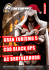### b c o p xx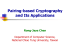### - hitechweb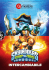### wired stereo headset for xbox / ps3 / pc / mac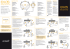### Télécharger - Thrustmaster